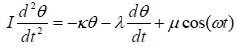# Solve Pendulum Eq'n: λ≠0, μ=0 | Angular Freq ωd & Eigenfreq ω0

• abotiz

#### abotiz

My assignment is in Swedish so I will try to Translate as good as I can ( if someone knows swedish you can visit the link http://physics.gu.se/~sjogren/FYP100/PM_svaengning.pdf )

## Homework Statement

We have a swinging Pendulum which equation is given by :The variables κ, λ and μ are positive constants which are characteristic of the swinging system. θ is the Pendulum angular deviation from the equilibrium position. I = inertia moment

The Question :

Solve the equation in 2 cases

Case 1 : λ=μ=0

Case 2 : λ≠0, μ=0

The Purpose :

Use the Solutions to derive a realtion between the eigenfrequency ω0, damping constant $$\gamma$$ and angular frequency ωd for the damping Oscillation.

2. The attempt at a solution

Many, and i do not know what to do, because the angular frequency ω = dθ/dt which make the term μ*cos(ω*t) the whole equation into a separable equation ? Iam confused

I need help with this one. A solution to this would be appreciated!

Thanks

Never mind.
Solved!